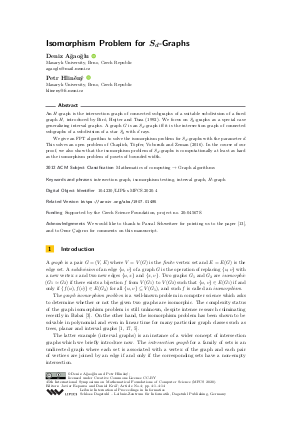Document# Isomorphism Problem for S_d-Graphs

### Authors Deniz Ağaoğlu, Petr Hliněný## File

LIPIcs.MFCS.2020.4.pdf
• Filesize: 0.52 MB
• 14 pages

## Acknowledgements

We would like to thank to Pascal Schweitzer for pointing us to the paper [Furst et al., 1980], and to Onur Çağırıcı for comments on this manuscript.

## Cite As

Deniz Ağaoğlu and Petr Hliněný. Isomorphism Problem for S_d-Graphs. In 45th International Symposium on Mathematical Foundations of Computer Science (MFCS 2020). Leibniz International Proceedings in Informatics (LIPIcs), Volume 170, pp. 4:1-4:14, Schloss Dagstuhl - Leibniz-Zentrum für Informatik (2020)
https://doi.org/10.4230/LIPIcs.MFCS.2020.4

## Abstract

An H-graph is the intersection graph of connected subgraphs of a suitable subdivision of a fixed graph H, introduced by Biró, Hujter and Tuza (1992). We focus on S_d-graphs as a special case generalizing interval graphs. A graph G is an S_d-graph iff it is the intersection graph of connected subgraphs of a subdivision of a star S_d with d rays. We give an FPT algorithm to solve the isomorphism problem for S_d-graphs with the parameter d. This solves an open problem of Chaplick, Töpfer, Voborník and Zeman (2016). In the course of our proof, we also show that the isomorphism problem of S_d-graphs is computationally at least as hard as the isomorphism problem of posets of bounded width.

## Subject Classification

##### ACM Subject Classification
• Mathematics of computing → Graph algorithms
##### Keywords
• intersection graph
• isomorphism testing
• interval graph
• H-graph

## Metrics

• Access Statistics
• Total Accesses (updated on a weekly basis)
0

## References

1. A. V. Aho, J. E. Hopcroft, and J. D. Ullman. The design and analysis of computer algorithms. Addison-Wesley, Reading, Mass. 1974, 1974.2. L. Babai. Monte carlo algorithms in graph isomorphism testing. Tech. Rep. 79-10, Université de Montréal, 1979. 42 pages.3. L. Babai. Graph isomorphism in quasipolynomial time [extended abstract]. In Proceedings of the 48th Annual ACM SIGACT Symposium on Theory of Computing, STOC 2016, pages 684-697. ACM, 2016. URL: https://doi.org/10.1145/2897518.2897542.
4. M. Biró, M. Hujter, and Z. Tuza. Precoloring extension. i. interval graphs. Discrete Mathematics 100, pages 267-279, 1992.5. K. S. Booth and G. S. Lueker. Testing for the consecutive ones property, interval graphs, and graph planarity using PQ-tree algorithms. J. Comput. Syst. Sci., 13:335-379, 1976.6. A. Bouland, A. Dawar, and E. Kopczynski. On tractable parameterizations of graph isomorphism. In Parameterized and Exact Computation, IPEC 2012. Proceedings, volume 7535 of Lecture Notes in Computer Science, pages 218-230. Springer, 2012. URL: https://doi.org/10.1007/978-3-642-33293-7_21.
7. S. Chaplick, M. Töpfer, J. Voborník, and P. Zeman. On H-topological intersection graphs. In WG, volume 10520 of Lecture Notes in Computer Science, pages 167-179. Springer, 2017.8. S. Chaplick and P. Zeman. Combinatorial problems on H-graphs. Electronic Notes in Discrete Mathematics, pages 61:223-229, 2017.9. F. R. K. Chung. On the cutwidth and the topological bandwidth of a tree. SIAM J. Alg. Discr. Meth., 6:268-277, 1985.10. R. Downey and M. Fellows. Fundamentals of Parameterized Complexity. Texts in Computer Science. Springer, 2013.11. P. A. Fejer and D. A. Simovici. Partially ordered sets. In: Mathematical Foundations of Computer Science. Texts and Monographs in Computer Science. Springer, New York, NY, 1991.12. F. V. Fomin, P. A. Golovach, and J. F. Raymond. On the tractability of optimization problems on H-graphs. In 26th Annual European Symposium on Algorithms, ESA 2018, volume 112 of LIPIcs, pages 30:1-30:14. Schloss Dagstuhl, 2018. URL: https://doi.org/10.4230/LIPIcs.ESA.2018.30.
13. M. L. Furst, J. E. Hopcroft, and E. M. Luks. Polynomial-time algorithms for permutation groups. 21st Annual Symposium on Foundations of Computer Science (FOCS), pages 36-41, 1980.14. M. L. Furst, J. E. Hopcroft, and E. M. Luks. A subexponential algorithm for trivalent graph isomorphism. In Proc. 11th Southeastern Conf. Combinatorics, Graph Theory, and Computing, Congressum Numerantium 3, 1980.15. F. Gavril. The intersection graphs of subtrees in trees are exactly the chordal graphs. Journal of Combinatorial Theory, Series B, 16:47-56, 1974.16. M. Grohe, D. Neuen, P. Schweitzer, and D. Wiebking. An improved isomorphism test for bounded-tree-width graphs. In 45th International Colloquium on Automata, Languages, and Programming, ICALP 2018, volume 107 of LIPIcs, pages 67:1-67:14. Schloss Dagstuhl, 2018. URL: https://doi.org/10.4230/LIPIcs.ICALP.2018.67.
17. J. E. Hopcroft and J. K. Wong. Linear time algorithm for isomorphism of planar graphs. In STOC, pages 172-184, 1974.18. T. Krawczyk. Testing isomorphism of circular-arc graphs - Hsu’s approach revisited. CoRR, abs/1904.04501, 2019. URL: http://arxiv.org/abs/1904.04501.
19. D. J. Rose, G. Lueker, and R. E. Tarjan. Algorithmic aspects of vertex elimination on graphs. SIAM Journal on Computing, 5:266–283, 1976.20. V. N. Zemlyachenko, N. M. Korneenko, and R. I. Tyshkevich. Graph isomorphism problem. J. of Soviet Mathematics, 29:1426-1481, 1985.X

Feedback for Dagstuhl Publishing